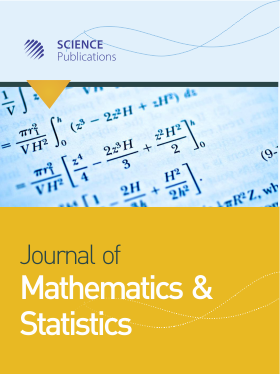Frequency: Continuous
ISSN: 1549-3644 (Print)
ISSN: 1558-6359 (Online)
Research Article Open Access

# A Measure of Monotonicity of two Random Variables

Farida Kachapova1 and Ilias Kachapov2
• 1 Auckland University of Technology, New Zealand
• 2 University of Auckland, New Zealand

## Abstract

Problem statement: When analyzing random variables it was useful to measure the degree of their monotone dependence or compare pairs of random variables with respect to their monotonicity. Existing coefficients measure general or linear dependence of random variables. Developing a measure of monotonicity was useful for practical applications as well as for general theory, since monotonicity was an important type of dependence. Approach: Existing measures of dependence are briefly reviewed. The Reimann coefficient was generalized to arbitrary random variables with finite variances. Results: The article describes criteria for monotone dependence of two random variables and introduces a measure of this dependence-monotonicity coefficient. The advantages of this coefficient are shown in comparison with other global measures of dependence. It was shown that the monotonicity coefficient satisfies natural conditions for a monotonicity measure and that it had properties similar to the properties of the Pearson correlation; in particular, it equals 1 (-1) if and only if the pair X, Y was comonotonic (counter-monotonic). The monotonicity coefficient was calculated for some bivariate distributions and the sample version of the coefficient was defined. Conclusion/Recommendations: The monotonicity coefficient should be used to compare pairs of random variables (such as returns from financial assets) with respect to their degree of monotone dependence. In the problems where the monotone relation of two variables has a random noise, the monotonicity coefficient can be used to estimate variance and other central moments of the noise. By calculating the sample version of the coefficient one will quickly find pairs of monotone dependent variables in a big dataset.

Journal of Mathematics and Statistics
Volume 8 No. 2, 2012, 221-228

DOI:

Submitted On: 6 March 2012 Published On: 26 May 2012

How to Cite: Kachapova, F. & Kachapov, I. (2012). A Measure of Monotonicity of two Random Variables. Journal of Mathematics and Statistics, 8(2), 221-228. https://doi.org/10.3844/jmssp.2012.221.228

• 1,737 Views
• 3,773 Downloads
• 2 Citations

## Download

### Keywords

• Monotonicity
• comonotonic
• counter-monotonic
• monotone dependence
• measure of dependence
• pearson correlation
• bivariate distributions
• random variables